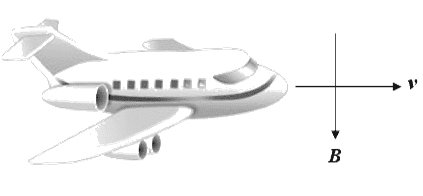# Plane immersed in the earth's vertically downward-pointing magnetic field

• syhpui2

## Homework Statement

This question and the next two refer to this situation:

http://i.imgur.com/dgJMR.pngA passenger jet is flying over Alaska in level flight at a constant altitude h = 10 km and constant speed of v = 300 m/s, immersed in the Earth's vertically downward-pointing magnetic field of B = 30 μT. The distance between the tips of the aircraft's metal wings is d = 50 m.

Part A:
Calculate the potential difference ε between the aircraft's wing tips due its motion through the Earth's magnetic field.
(a) ε = 0.0 volts
(b) ε = 0.45 volts
(c) ε = 0.90 volts

Part B
Which wingtip has positive charge, as viewed by a passenger in the airplane who is facing in the direction that the airplane is traveling?
(a) the right wingtip
(b) the left wingtip
(c) neither wingtip

## Homework Equations

emf = d flux / dt

flux = int[B*dA]

## The Attempt at a Solution

For part A, I tried formula above, I get answer C, because I thought I need to calculate difference for both wings? Part B I used F=BIL, however, I get the force is on right wing? What is the relationship between force ans its direction of the plane that traveling?

Thx